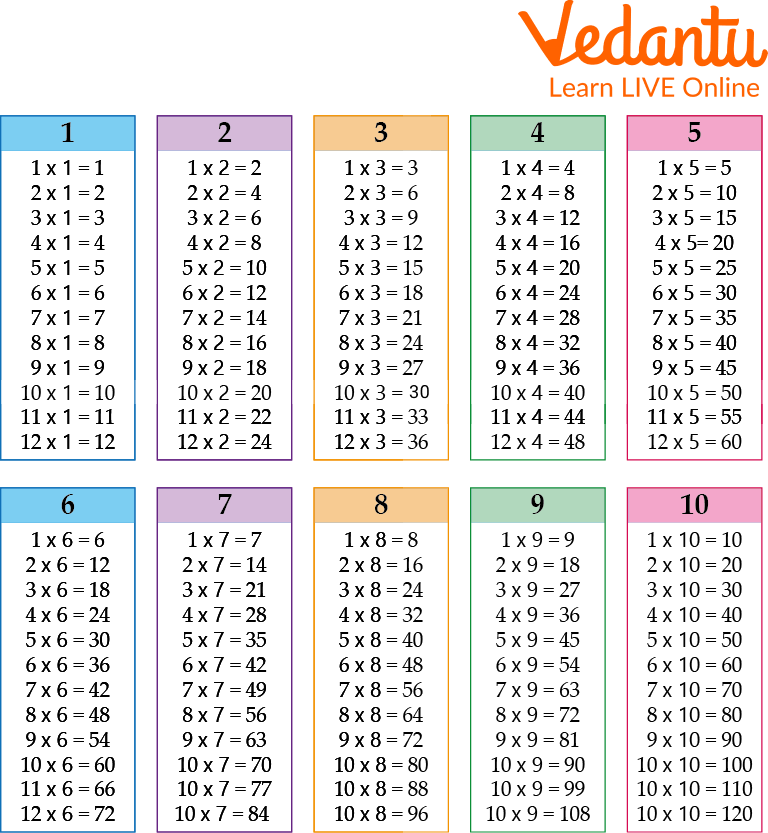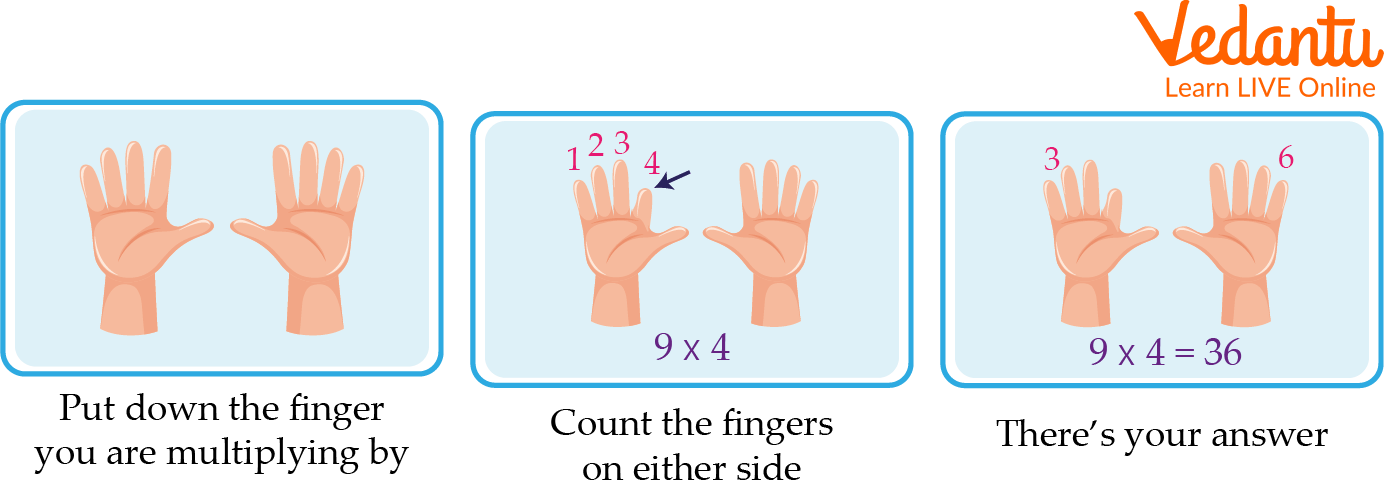Courses
Courses for Kids
Free study material
Free LIVE classes
More

# Multiplication Table Chart for KidsLIVE
Join Vedantu’s FREE Mastercalss

## Overview of Multiplication Table Chart

A multiplication table chart shows tables clearly in one grid. It's a handy reference to memorise multiplication facts. A multiplication chart is a table that shows the product of two numbers. Usually, one set of numbers is written on the left column and another on the topmost row. A multiplication chart saves a lot of time and energy in doing calculations.

The first use of a maths table is unknown, but they have been used since at least the 19th century. Maths tables are essential for understanding mathematics. The students are advised to learn Maths tables else it would be difficult to understand the other aspects of mathematics. Let's start with the multiplication table chart for kids.

## Multiplication Tables Chart

A multiplication table chart is a list of multiples of a number. We can get the multiplication table by multiplying the given number with whole numbers. Multiplication is one of the basic mathematical operations which is taught to students at an early age. A simple way to teach students the concept of multiplication is through a multiplication table chart.

Times tables can be very helpful in doing basic mathematical calculations. These work as building blocks for higher maths like fractions, decimals, factors and many more. Table charts for kids are provided to help you learn times tables by heart.Times Tables Chart

## Importance of Tables in Mathematics

The following are the importance of tables:

• Learning times tables make our work easier. We can make fast calculations.

• It helps us in everyday life. It makes maths more interesting.

• Maths tables are very useful and will help students learn further chapters of algebra.

• They are very helpful in exams and calculations as they work as a time saver. Long calculations can be solved in seconds.

• The importance of tables is not only in Mathematics but also in everyday life. They build good communication skills and improve mental maths. This is how tables play an important role in Mathematics.

## How to Make Kids Learn Tables?

Most experts think that kids begin learning tables around the age of 6. This is when they become more aware of their numbers and understand how to work with them. The method of teaching tables to children varies with the understanding and grasping capabilities of the child. Here are some points which will help you to understand how to make kids learn tables faster:

• The Waldorf multiplication flower.

• A times table sheet in the classroom.

• Explaining addition facts is an effective way to teach multiplication tables.How to Make Kids Learn Tables?

## What is the Objective of Learning Tables?

One of the objectives of learning tables is to develop an understanding of multiplication and division. This can be achieved by learning the table facts and also by using tables to help solve problems. Tables can also help understand other concepts such as fractions, decimals, multiples, and factors.

## Solved Examples

Q 1. A bicycle costs Rs.200. How much will be paid for 8 such bicycles?

Ans: The cost of one bicycle = 200 Rs

Number of bicycles = 8

The cost of 8 bicycles = 200 × 8

= 1600 Rs

Thus 8 bicycles cost 1600 Rs.

Q 2. Multiply 3014 by 2.

Ans:

Step 1: Start with the digit in ones place. (2 × 4 = 8)

Step 2: Multiply 2 with the digit in the tens place. (2 × 1 = 2)

Step 3: Now, multiply 2 with the digit in the hundreds place. (2 × 0 = 0)

Step 4: Now multiply 2 with the digit in the thousands place. (2 × 3 = 6)

Step 5: 3014 × 2 = 6028.

Q 3 Sam works for 5 hours a day and is paid \$8 per hour. How much does Sam earn in a day?

Ans: Sam works for 5 hours and is paid \$8 per hour. Therefore, by using multiplication tables, the total amount he earns is 5 times 8.

Therefore, Sam earns \$40.

## Practice Questions

Q 1 Ram has 6 chocolates. He shares them equally with his 3 friends. How many chocolates does each friend get?

Ans: Each friend gets 2 chocolates.

Q 2 What is 784 times 44?

Ans: 784 times 44 is 34496.

## Summary

A great way to learn your timetables. They help you to understand how multiplication works and can be a fun way to learn your tables. There are many different ways to learn your timetables, but a Maths times table is a great way to start. We have discussed how to make kids learn tables on their tips by using tricks in the chapter. Table charts for kids make our calculations easier and faster. So learning multiplication table charts is helpful in great ways.

Last updated date: 02nd Oct 2023
Total views: 78.3k
Views today: 0.78k

## FAQs on Multiplication Table Chart for Kids

1. Why is a multiplication chart helpful?

A multiplication chart shows all the times tables in one grid. Create a Math multiplication table chart, read it daily, or utilise it for calculation purposes. This will help students memorise it.

2. Who invented multiplication?

The Babylonians invented the multiplications.

3. What is the purpose of multiplication?

Multiplication helps us find the total number of items quickly. For multiplication, we will consider the number of equal-sized groups and the number of items in each group.